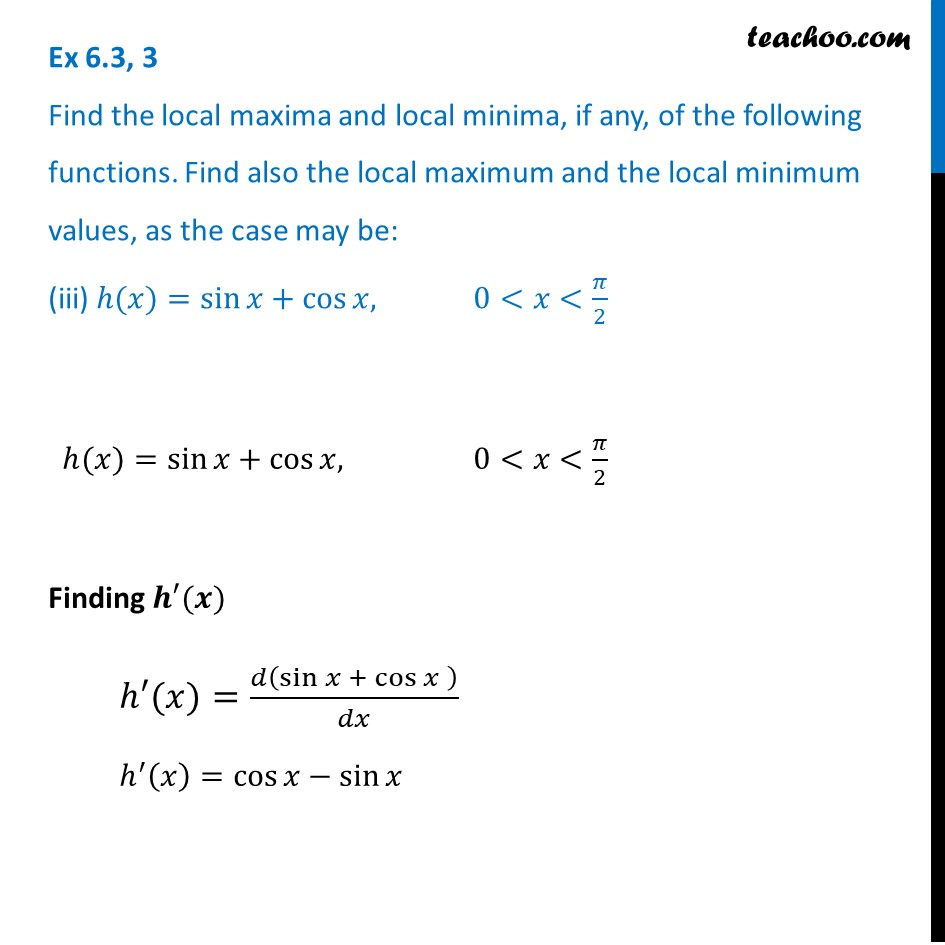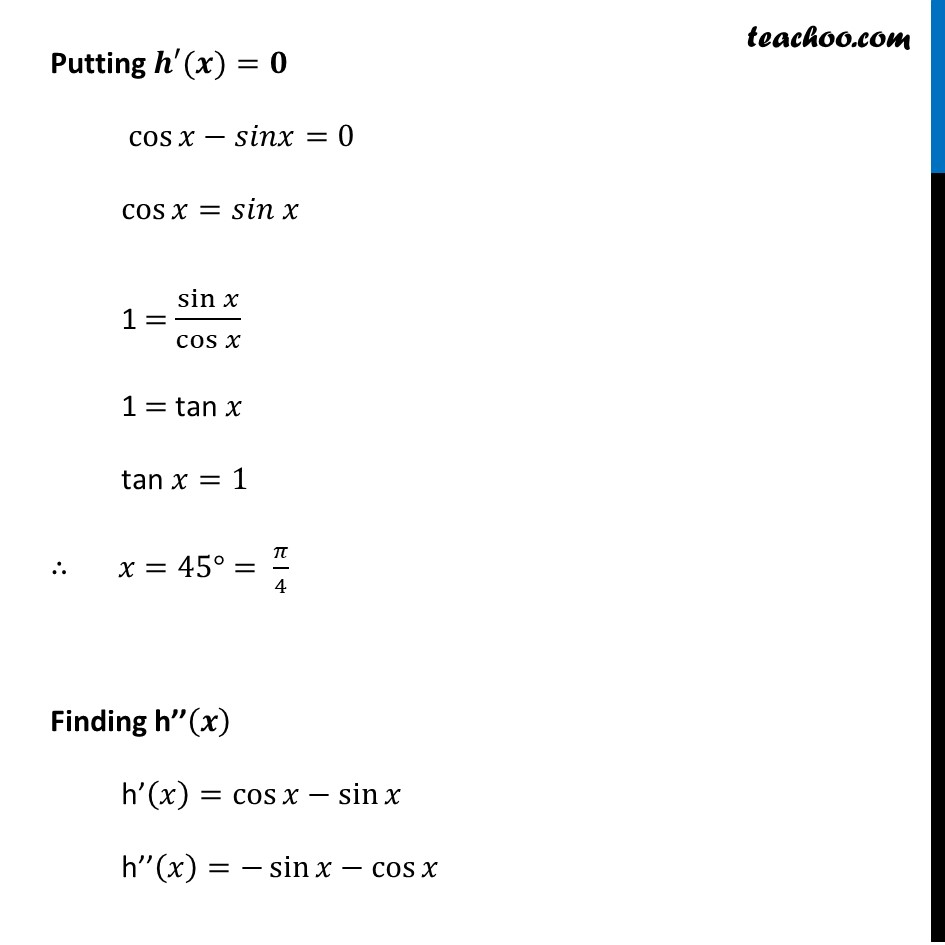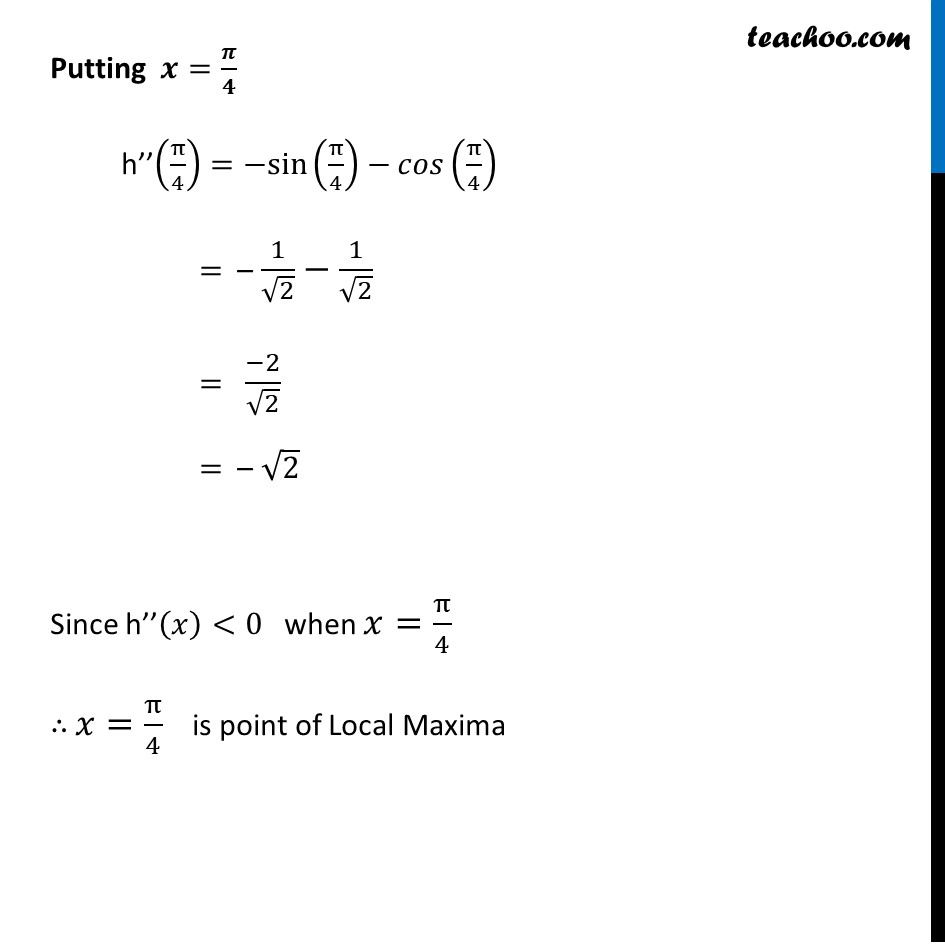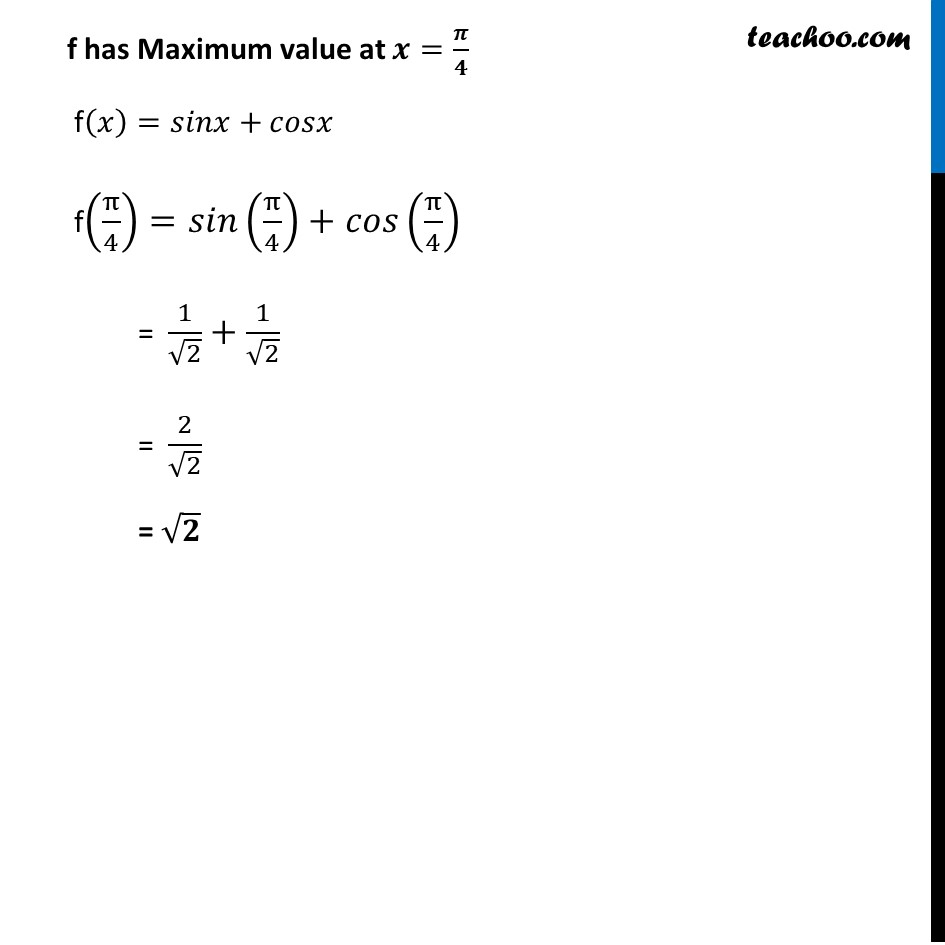Ex 6.3

Chapter 6 Class 12 Application of Derivatives
Serial order wiseLearn in your speed, with individual attention - Teachoo Maths 1-on-1 Class

### Transcript

Ex 6.3, 3 Find the local maxima and local minima, if any, of the following functions. Find also the local maximum and the local minimum values, as the case may be: (iii) ℎ(𝑥)=sin⁡𝑥+cos⁡𝑥, 0<𝑥<𝜋/2 ℎ(𝑥)=sin⁡𝑥+cos⁡𝑥, 0<𝑥<𝜋/2 Finding 𝒉′(𝒙) ℎ′(𝑥)=𝑑(sin⁡𝑥 + cos⁡𝑥" " )/𝑑𝑥 ℎ^′ (𝑥)=cos⁡𝑥−sin⁡𝑥 Putting 𝒉′(𝒙)=𝟎 cos⁡𝑥−𝑠𝑖𝑛𝑥=0 cos⁡〖𝑥=𝑠𝑖𝑛 𝑥〗 1 = sin⁡𝑥/cos⁡𝑥 1 = tan 𝑥 tan 𝑥=1 ∴ 𝑥=45°= 𝜋/4 Finding h’’(𝒙) h’(𝑥)=cos⁡𝑥−sin⁡𝑥 h’’(𝑥)=−sin⁡𝑥−cos⁡𝑥 Putting 𝒙=𝝅/𝟒 h’’(π/4)=−sin(π/4)−𝑐𝑜𝑠(π/4) = – 1/√2−1/√2 = (−2)/√2 = – √2 Since h’’(𝑥)<0 when 𝑥=π/4 ∴ 𝑥=π/4 is point of Local Maxima f has Maximum value at 𝒙=𝝅/𝟒 f(𝑥)=𝑠𝑖𝑛𝑥+𝑐𝑜𝑠𝑥 f(π/4)=𝑠𝑖𝑛(π/4)+𝑐𝑜𝑠(π/4) = 1/√2+1/√2 = 2/√2 = √𝟐